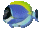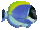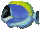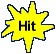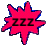Solve Equations Types:   ay+b=c  ay-b=c       Math Fun Game Tips:

- To solve 2y+7 = 19, the first step is to isolate the term 2y by removing the +7 from the left side.
To remove +7 from 2y+7, you use the inverse of adding 7, namely subtracting 7 from each side.
The first step can be written as 2y+7-7 = 19-7 .

The second step simplifies each side of the equation and is written as 2y = 12 .

The third step uses the method 2y/2 = 12/2 to isolate the variable y on one side.

The final step isolates the y by simplifying each side of the equation and is written as y = 6.

- For equation 2y+7 = 19, the answer y=6 is the value of the variable y that satisfies the equation.
To satisfy an equation means to make its left side value equal its right side value.
When you check the answer y=6, the left side value of 2y+7 evaluates as 2(6)+7 which is 19.
When you check the right side value of 2y+7 = 19, it is already 19 with no evaluation work needed.

- The answer y=6 that satisfies the equation 2y+7 = 19 can be called the solution or root.

- Similarly, a complete solution for solving an equation such as 3w-8 = 22 to find the value of w is ...
3w-8 = 22
3w-8+8 = 22+8
3w = 30
3w/3 = 30/3
w = 10

- Your Game Score is reduced by the number of fish hits.

- To slow the game speed repeat tap/click on the word Slider.
- To increase the game speed repeat tap/click on the word Math.
- Speed can also be adjusted with a keyboard's - and + keys.

- Refresh/Reload the web page to restart the game.